TitleCollege Algebra
Tutorial 54B:
SeriesLearning Objectives

 After completing this tutorial, you should be able to: Know how to evaluate a summation. Find the sum of a series. Write a series in summation notation. Find the mean of a sequence of numbers.Introduction

 In this tutorial we will mainly be going over series.  We will start by going through some basic terminology associated with series.  In a series you are working with the sum of terms of a sequence.  If you need a review on sequences, feel free to go to Tutorial 54A: Sequences.  Arithmetic and geometric series are special forms that are looked at more in depth in Tutorial 54C: Arithmetic Sequences and Series and Tutorial 54D: Geometric Sequences and Series.  We will be looking at series forwards and backwards.  Once you are able to go back and forth, then that means you have series down.  Enough of that, let's get started.Tutorial

 Summation Notation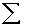The greek letter sigma is used to indicate a summation. Summation notation is a shorthand way of saying take the sum of certain terms of a sequence. Let’s look at the following:In this example, i represents the term number or index of summation.  Note that any variable can be used here.represents the general term. 1 represents the lower limit of summation and n represents the upper limit of summation.  Note that these numbers can be any integer. Basically, you will find the sum of the terms that start at the lower limit and go through the upper limit. This expression would represent  the sum of terms 1 through n: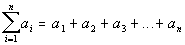Series

 A series is a sum of terms of a sequence. It can be written in summation notation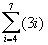or expanded out in a sum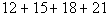. If you need a review on sequences, feel free to go to Tutorial 54A: Sequences.

 Finite Series

 A finite series is a series that has a sum of a finite number of terms. All the examples above are finite series.

 Infinite Series

 An infinite series is a series that has a sum of an infinite number of terms. In a infinite series, the upper limit is infinity ... which means there is no upper bound. An example of an infinite series is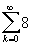or it can be written like. The three dots indicate that this pattern will keep continuing on and on.Example 1: Find the sum of the series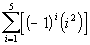.

You find the terms of the series in the same fashion that you do for a sequence.  Plug the term number in for the given variable.  So in this problem, wherever there is an i in the term, the term number will replace it.

If you need a review on sequences, feel free to go to Tutorial 54A: Sequences.

The difference between this problem and a sequence problem is that you will be adding all of the terms together to get your end result.

What values will you be plugging in to get the terms that will be summed?

If you said 1 through 5 you are right on!!!

Let’s see what we get when we add up terms plugging in 1, 2, 3, 4, and 5 for i:Example 2: Find the sum of the series.

You find the terms of the series in the same fashion that you do for a sequence.  Plug the term number in for the given variable.  So in this problem, wherever there is an n in the term, the term number will replace it.

If you need a review on sequences, feel free to go to Tutorial 54A: Sequences.

Also note that this problem has a factorial in it.  If you need a review on factorials, feel free to go to Tutorial 54A: Sequences.

The difference between this problem and a sequence problem is that you will be adding all of the terms together to get your end result.

What values will you be plugging in to get the terms that will be summed?

If you said 0 through 6 you are right on!!!

Let’s see what we get when we add up terms plugging in 0, 1, 2, 3, 4, 5, and 6 for n:Example 3: Find the sum of the series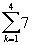.

You find the terms of the series in the same fashion that you do for a sequence.  Plug the term number in for the given variable.  So in this problem, wherever there is an k in the term, the term number will replace it.

If you need a review on sequences, feel free to go to Tutorial 54A: Sequences.

The difference between this problem and a sequence problem is that you will be adding all of the terms together to get your end result.

What values will you be plugging in to get the terms that will be summed?

If you said 1 through 4 you are right on!!!

Let’s see what we get when we add up terms plugging in 1, 2, 3, and 4 for k: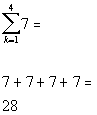Note that the general term is 7, which is a constant.  So no matter what k is, the term is always 7.  Since we were going from 1 to 4, we had 4 terms of 7 be added or 4(7) = 28.

In general, if your general term is constant than the sum will end up being the number of terms in your series times the constant.Example 4: Write the series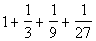in summation notation.  Use the index i and let i begin with 1.

Again, this is similar to working with sequences.  You need to analyze what is happening term by term and see how it relates to the index number.

One thing that is always constant is that the numerator of each term is 1.

However the denominator changes.

We need to figure out the relationship between i and the denominator:

 When i is 1, the denominator is 1 When i is 2, the denominator is 3. When i is 3, the denominator is 9. When i is 4, the denominator is 27.

What do you think the relationship is?
It looks like the denominator is always 3 to a power.  It also looks like it starts as 3 to the 0 power, then 3 to 1 power, and so forth.  Since we have to start with i = 1, we will have to adjust the power so that it will start with 0.

So how will we represent the power?
If you said i - 1, give yourself a pat on the back.

Putting it together,  we can write the summation as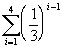An equivalent way to write this is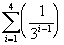.

Sometimes you have to play around with it before you get it just right.  You can always check it by putting in the i values and seeing if you get the given series.

This one does check.Example 5: Write the seriesin summation notation.  Use the index i and let i begin with 1.

Again, this is similar to working with sequences.  You need to analyze what is happening term by term and see how it relates to the index number.

One thing that is always constant is that each term contains an e.

There are also two things that change.

First let's look at the alternating signs:

 The first term is positive, the second term is negative, the third positive, the fourth negative and so forth. When i is odd (1, 3, 5, ...), then the term is positive. When i is even (2, 4, 6, ...), then the term is negative.  So do you think we are going to haveor. If you saidyou are correct!!!  If n is odd, then this term will be positive.  If n is even, then this term will be negative.

Next, let’s look at the exponent on each term.

We need to figure out the relationship between i and the exponent:

 When i is 1, the exponent is 3. When i is 2, the exponent is 4. When i is 3, the exponent is 5. When i is 4, the exponent is 6. And so forth....

What do you think the relationship is?

So how will we represent the power?
If you said i + 2, give yourself a pat on the back.

Putting it together,  we can write the summation as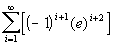Sometimes you have to play around with it before you get it just right.  You can always check it by putting in the i values and seeing if you get the given series.

This one does check.Example 6: Rewrite the seriesusing the new index j such that=.

We need to rewrite the series so that they are both equivalent to each other, but the first one starts at n = 2 and the second one starts at j = 0.

We need to find the relationship between n and j and rewrite n in terms of j:
Looking at the lower limits for each, how would you write n in terms of j?  What is their relationship?

If you said n = j + 2, you are correct!!!

Basically, we need to do a substitution.  Wherever we have an n in our general term, we will replace it with j + 2:Mean of a Sequence of Numbers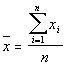When you find a mean of a set of numbers, you add up all of the numbers and divide it by the number of values that you have.  You are doing the same thing here.  This is just a special set of numbers that come from a sequence.Example 7: Find the mean of the sequence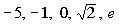.

 Note that I will be rounding the square root of 2 to 1.414 and e to 2.718. We need to find the mean, so we will be adding all of the values that we have and dividing that sum by what number? If you said 5, you are correct! Let’s see what we get: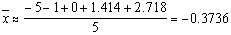Practice Problems

 These are practice problems to help bring you to the next level.  It will allow you to check and see if you have an understanding of these types of problems. Math works just like anything else, if you want to get good at it, then you need to practice it.  Even the best athletes and musicians had help along the way and lots of practice, practice, practice, to get good at their sport or instrument.  In fact there is no such thing as too much practice. To get the most out of these, you should work the problem out on your own and then check your answer by clicking on the link for the answer/discussion for that  problem.  At the link you will find the answer as well as any steps that went into finding that answer.Practice Problems 1a - 1b: Find the sum of the given series.

 1a.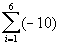(answer/discussion to 1a) 1b.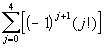(answer/discussion to 1b)Practice Problem 2a: Write the series in summation notation.  Use the index i and let i begin with 1.

 2a.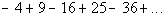(answer/discussion to 2a)Practice Problem 3a: Rewrite the series using the new index j as indicated.

 3a.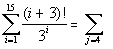(answer/discussion to 3a)Practice Problem 4a: Find the mean of the sequence.

 4a.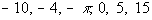(answer/discussion to 4a)Need Extra Help on these Topics?

 There were no good websites found to help us with the topics on this page.   Go to Get Help Outside the Classroom found in Tutorial 1: How to Succeed in a Math Class for some more suggestions.

Last revised on May 17, 2011 by Kim Seward.